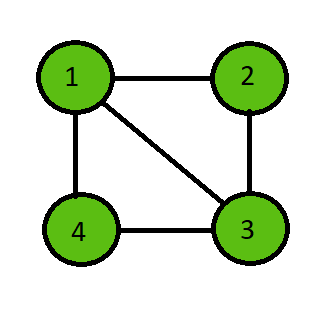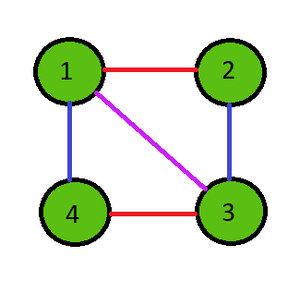# Java Program to Implement the Vizing’s Theorem

• Last Updated : 22 Feb, 2021

Vizing’s Theorem of graph theory states that every simple undirected graph has a chromatic index of one larger than the maximum degree ‘d’ of the graph. In simple meaning, the theorem states that the chromatic index can be either ‘d’ or ‘d’+1.

Chromatic Index of a graph is the minimum number of colors required to color the edges of the graph such that any two edges that share the same vertex have different colors.

Examples:

Input:Output:

Chromatic Index = 3

Edge from 1 to 2 : Color 1

Edge from 2 to 3 : Color 2

Edge from 3 to 4 : Color 1

Edge from 4 to 1 : Color 2

Edge from 1 to 3 : Color 3Algorithm:

Below is the step-by-step approach of the algorithm:-

• Initialize the number of edges and the edge list.
• Color the graph according to the Vizing’s Theorem.
• Assign a color to an edge and check if any adjacent edges have the same color or not.
• If any adjacent edge has the same color, then increment the color to try the next color for that edge.
• Repeat till all the edges get it’s color according to the theorem.
• Once done print the maximum value of color for all the edges and the colors of every edge.

Implementation of the above approach:

## Java

 `// Java program to Implement``// Vizing's Theorem`` ` `import` `java.util.*;`` ` `public` `class` `chromaticIndex {`` ` `    ``// Function to find the chromatic index``    ``public` `void` `edgeColoring(``int``[][] edges, ``int` `e)``    ``{``        ``// Initialize edge to first``        ``// edge and color to color 1``        ``int` `i = ``0``, color = ``1``;`` ` `        ``// Repeat until all edges are done coloring``        ``while` `(i < e) {`` ` `            ``// Give the selected edge a color``            ``edges[i][``2``] = color;`` ` `            ``boolean` `flag = ``false``;`` ` `            ``// Iterate through all others edges to check``            ``for` `(``int` `j = ``0``; j < e; j++) {`` ` `                ``// Ignore if same edge``                ``if` `(j == i)``                    ``continue``;`` ` `                ``// Check if one vertex is similar``                ``if` `((edges[i][``0``] == edges[j][``0``])``                    ``|| (edges[i][``1``] == edges[j][``0``])``                    ``|| (edges[i][``0``] == edges[j][``1``])``                    ``|| (edges[i][``1``] == edges[j][``1``])) {`` ` `                    ``// Check if color is similar``                    ``if` `(edges[i][``2``] == edges[j][``2``]) {`` ` `                        ``// Increment the color by 1``                        ``color++;``                        ``flag = ``true``;``                        ``break``;``                    ``}``                ``}``            ``}`` ` `            ``// If same color faced then repeat again``            ``if` `(flag == ``true``) {``                ``continue``;``            ``}`` ` `            ``// Or else proceed to a new vertex with color 1``            ``color = ``1``;``            ``i++;``        ``}`` ` `        ``// Check the maximum color from all the edge colors``        ``int` `maxColor = -``1``;``        ``for` `(i = ``0``; i < e; i++) {``            ``maxColor = Math.max(maxColor, edges[i][``2``]);``        ``}`` ` `        ``// Print the chromatic index``        ``System.out.println(``"Chromatic Index = "` `+ maxColor);``       ` `        ``for` `(i = ``0``; i < e; i++)``        ``{``            ``System.out.println(``"Edge from "` `+ edges[i][``0``]``                               ``+ ``" to "` `+ edges[i][``1``]``                               ``+ ``" : Color "` `+ edges[i][``2``]);``        ``}``    ``}`` ` `    ``// Driver code``    ``public` `static` `void` `main(String[] args)``    ``{`` ` `        ``// Number of edges``        ``int` `e = ``5``;`` ` `        ``// Edge list``        ``int``[][] edges = ``new` `int``[e][``3``];`` ` `        ``// Initialize all edge colors to 0``        ``for` `(``int` `i = ``0``; i < e; i++) {``            ``edges[i][``2``] = -``1``;``        ``}`` ` `        ``// Edges``        ``edges[``0``][``0``] = ``1``;``        ``edges[``0``][``1``] = ``2``;`` ` `        ``edges[``1``][``0``] = ``2``;``        ``edges[``1``][``1``] = ``3``;`` ` `        ``edges[``2``][``0``] = ``3``;``        ``edges[``2``][``1``] = ``4``;`` ` `        ``edges[``3``][``0``] = ``4``;``        ``edges[``3``][``1``] = ``1``;`` ` `        ``edges[``4``][``0``] = ``1``;``        ``edges[``4``][``1``] = ``3``;`` ` `        ``// Run the function``        ``chromaticIndex c = ``new` `chromaticIndex();``        ``c.edgeColoring(edges, e);``    ``}``}`
Output
```Chromatic Index = 3
Edge from 1 to 2 : Color 1
Edge from 2 to 3 : Color 2
Edge from 3 to 4 : Color 1
Edge from 4 to 1 : Color 2
Edge from 1 to 3 : Color 3
```

My Personal Notes arrow_drop_up